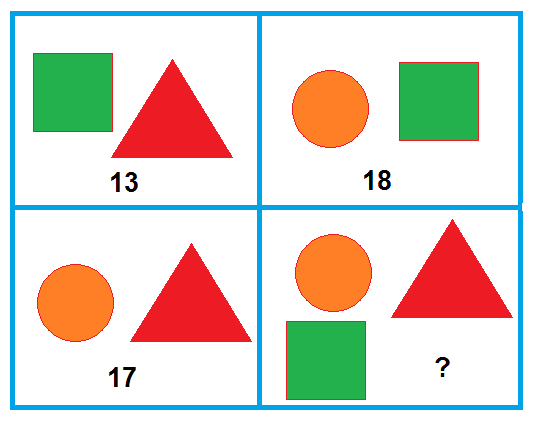# We Only Know Two Of Each!

Algebra Level 1If the numbers in each box represent the total weight of the figures respectively, then which number should replace the question mark?

×